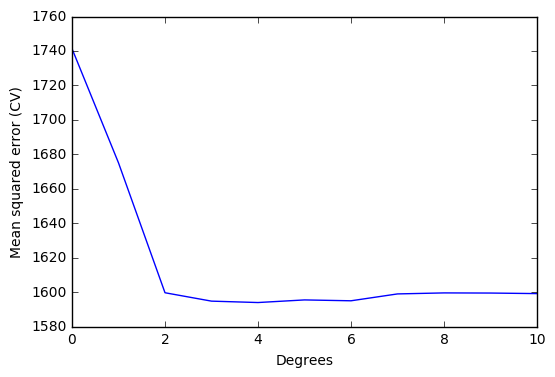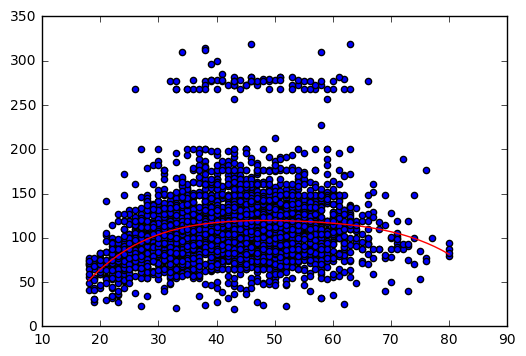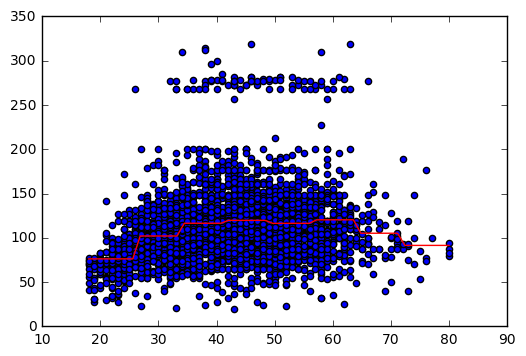# Exercise 7.6

import pandas as pd
import numpy as np
import matplotlib.pyplot as plt
import statsmodels.api as sm
import statsmodels.formula.api as smf
from sklearn.pipeline import Pipeline
from sklearn.linear_model import LinearRegression
from sklearn.preprocessing import PolynomialFeatures
from sklearn import metrics
from sklearn.model_selection import cross_val_score
from sklearn.feature_selection import f_regression

%matplotlib inline

df = pd.read_csv('../data/Wage.csv', index_col=0)

df.head()

year age sex maritl race education region jobclass health health_ins logwage wage
231655 2006 18 1. Male 1. Never Married 1. White 1. < HS Grad 2. Middle Atlantic 1. Industrial 1. <=Good 2. No 4.318063 75.043154
86582 2004 24 1. Male 1. Never Married 1. White 4. College Grad 2. Middle Atlantic 2. Information 2. >=Very Good 2. No 4.255273 70.476020
161300 2003 45 1. Male 2. Married 1. White 3. Some College 2. Middle Atlantic 1. Industrial 1. <=Good 1. Yes 4.875061 130.982177
155159 2003 43 1. Male 2. Married 3. Asian 4. College Grad 2. Middle Atlantic 2. Information 2. >=Very Good 1. Yes 5.041393 154.685293
11443 2005 50 1. Male 4. Divorced 1. White 2. HS Grad 2. Middle Atlantic 2. Information 1. <=Good 1. Yes 4.318063 75.043154

# (a)

# Model variables
y = df['wage'][:,np.newaxis]
X = df['age'][:,np.newaxis]

# Compute regression models with different degrees
# Variable 'scores' saves mean squared errors resulting from the different degrees models.
# Cross validation is used
scores = []
for i in range(0,11):
model = Pipeline([('poly', PolynomialFeatures(degree=i)), ('linear', LinearRegression())])
model.fit(X,y)

score = cross_val_score(model, X, y, cv=5, scoring='neg_mean_squared_error')
scores.append(np.mean(score))

scores = np.abs(scores)  # Scikit computes negative mean square errors, so we need to turn the values positive.

# Plot errors
x_plot = np.arange(0,11)

plt.plot(x_plot, scores)
plt.ylabel('Mean squared error (CV)')
plt.xlabel('Degrees')
plt.xlim(0,10)
plt.show()# Print array element correspoding to the minimum mean squared error
# Element number = polynomial degree for minimum mean squared error
print(np.where(scores == np.min(scores)))

(array(, dtype=int64),)


Optimal degree d for the polynomial according to cross-validation: 4

We will use statsmodels to perform the hypothesis testing using ANOVA. Statsmodels has a built-in function that simplifies our job and we didn't find an equivalent way of solving the problem with scikit-learn.

# Fit polynomial models to use in statsmodels.
models=[]
for i in range(0,11):
poly = PolynomialFeatures(degree=i)
X_pol = poly.fit_transform(X)
model = smf.GLS(y, X_pol).fit()
models.append(model)

# Hypothesis testing using ANOVA
sm.stats.anova_lm(models, models, models, models, models, models, models, typ=1)

df_resid ssr df_diff ss_diff F Pr(>F)
0 2999.0 5.222086e+06 0.0 NaN NaN NaN
1 2998.0 5.022216e+06 1.0 199869.664970 125.505882 1.444930e-28
2 2997.0 4.793430e+06 1.0 228786.010128 143.663571 2.285169e-32
3 2996.0 4.777674e+06 1.0 15755.693664 9.893609 1.674794e-03
4 2995.0 4.771604e+06 1.0 6070.152124 3.811683 5.098933e-02
5 2994.0 4.770322e+06 1.0 1282.563017 0.805371 3.695646e-01
6 2993.0 4.766389e+06 1.0 3932.257136 2.469216 1.162015e-01

The lower the values of F, the lower the significance of the coefficient. Degrees higher than 4 don't improve the polynomial regression model significantly. This results is in agreement with cross validation results.

# Save optimal degree
opt_degree = 4

# Plot polynomial regression
# Auxiliary variables X_line and y_line are created.
# These variables allow us to draw the polynomial regression.
# np.linspace() is used to create an ordered sequence of numbers. Then we can plot the polynomial regression.
model = Pipeline([('poly', PolynomialFeatures(degree = opt_degree)), ('linear', LinearRegression())])
model.fit(X,y)

X_lin = np.linspace(18,80)[:,np.newaxis]
y_lin = model.predict(X_lin)

plt.scatter(X,y)
plt.plot(X_lin, y_lin,'-r');# (b)

# Compute cross-validated errors of step function
'''
To define the step function, we need to cut the dataset into parts (pd.cut() does the job)
and associate a each part to a dummy variable. For example, if we have two parts (age<50
and age >= 50), we will have one dummy variable that gets value 1 if age<50 and value 0
if age>50.

Once we have the dataset in these conditions, we need to fit a linear regression to it.
The governing model will be defined by: y = b0 + b1 C1 + b2 C2 + ... + bn Cn, where
b stands for the regression coefficient;
C stands for the value of a dummy variable.

Using the same example as above, we have y = b0 + b1 C1, thus ...
'''
scores = []
for i in range(1,10):
age_groups = pd.cut(df['age'], i)
df_dummies = pd.get_dummies(age_groups)

X_cv = df_dummies
y_cv = df['wage']

model.fit(X_cv, y_cv)
score = cross_val_score(model, X_cv, y_cv, cv=5, scoring='neg_mean_squared_error')
scores.append(score)

scores = np.abs(scores)  # Scikit computes negative mean square errors, so we need to turn the values positive.

# Number of cuts that minimize the error
min_scores = []
for i in range(0,9):
min_score = np.mean(scores[i,:])
min_scores.append(min_score)

print('Number of cuts: %i, error %.3f' % (i+1, min_score))

Number of cuts: 1, error 1741.335
Number of cuts: 2, error 1733.925
Number of cuts: 3, error 1687.688
Number of cuts: 4, error 1635.756
Number of cuts: 5, error 1635.556
Number of cuts: 6, error 1627.397
Number of cuts: 7, error 1619.168
Number of cuts: 8, error 1607.926
Number of cuts: 9, error 1616.550


The number of cuts that minimize the error is 8.

# Plot
# The following code shows, step by step, how to plot the step function.

# Convert ages to groups of age ranges
n_groups = 8
age_groups = pd.cut(df['age'], n_groups)

# Dummy variables
# Dummy variables is a way to deal with categorical variables in linear regressions.
# It associates the value 1 to the group to which the variable belongs, and the value 0 to the remaining groups.
# For example, if age == 20, the (18,25] will have the value 1 while the group (25, 32] will have the value 0.
age_dummies = pd.get_dummies(age_groups)

# Dataset for step function
# Add wage to the dummy dataset.
df_step = age_dummies.join(df['wage'])

df_step.head()  # Just to visualize the dataset with the specified number of cuts

(17.938, 25.75] (25.75, 33.5] (33.5, 41.25] (41.25, 49] (49, 56.75] (56.75, 64.5] (64.5, 72.25] (72.25, 80] wage
231655 1.0 0.0 0.0 0.0 0.0 0.0 0.0 0.0 75.043154
86582 1.0 0.0 0.0 0.0 0.0 0.0 0.0 0.0 70.476020
161300 0.0 0.0 0.0 1.0 0.0 0.0 0.0 0.0 130.982177
155159 0.0 0.0 0.0 1.0 0.0 0.0 0.0 0.0 154.685293
11443 0.0 0.0 0.0 0.0 1.0 0.0 0.0 0.0 75.043154
# Variables to fit the step function
# X == dummy variables; y == wage.
X_step = df_step.iloc[:,:-1]
y_step = df_step.iloc[:,-1]

# Fit step function (statsmodels)
reg = sm.GLM(y_step[:,np.newaxis], X_step).fit()

# Auxiliary data to plot the step function
# We need to create a comprehensive set of ordered points to draw the figure.
# These points are based on 'age' values but include also the dummy variables identifiying the group that 'age' belongs.
X_aux = np.linspace(18,80)
groups_aux = pd.cut(X_aux, n_groups)
aux_dummies = pd.get_dummies(groups_aux)

# Plot step function
X_step_lin = np.linspace(18,80)
y_lin = reg.predict(aux_dummies)

plt.scatter(X,y)
plt.plot(X_step_lin, y_lin,'-r');• http://statsmodels.sourceforge.net/stable/examples/notebooks/generated/glm_formula.html
• http://pandas.pydata.org/pandas-docs/stable/generated/pandas.cut.html